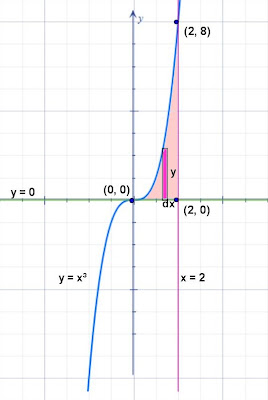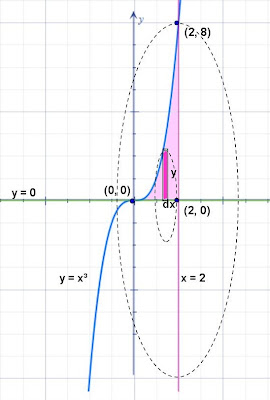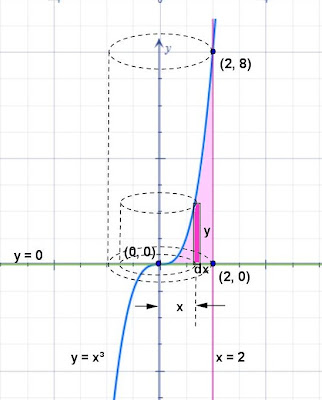## Wednesday, April 3, 2013

### Volume - Solid Revolution

Category: Integral Calculus, Analytic Geometry

"Published in Newark, California, USA"

Find the volume generated by revolving about the x-axis and y-axis the areas bounded by the curves

Solution:

To illustrate the problem, it is better to sketch the graph of the three equations above using the principles of Analytic Geometry as followsPhoto by Math Principles in Everyday Life

From the figure above, the point of intersection between y = x3 and y = 0 is (0, 0), the point of intersection between y = 0 and x = 2 is (2, 0), and the point of intersection between y = x3 and x = 2 is (2, 8) by substituting x = 2 to y = x3

Next, from the given three equations above, it is better to use a vertical strip at the area bounded by three curves and label further the figure as followsPhoto by Math Principles in Everyday Life

If you rotate the shaded area about the x-axis, the vertical strip becomes a disk as followsPhoto by Math Principles in Everyday Life

The volume of a disk formed by the rotation of a vertical strip about the x-axis is

Integrate on both sides of the equation to get the volume of a solid formed by the rotation of the area about the x-axis as follows

If you rotate the shaded area about the y-axis, the vertical strip becomes a cylindrical shell as followsPhoto by Math Principles in Everyday Life

The volume of a cylindrical shell formed by the rotation of a vertical strip about the y-axis is

The circumference of the base of the cylindrical shell is 2πx. x is the distance of the vertical strip to the axis of revolution which is the y-axis. y is the height of a cylindrical shell. dx is the thickness of a cylindrical shell. Since dx is a very small value, then the two radii of the cylindrical shell are almost the same which is x. The three dimensions of a thin rectangular box are 2πx, y, and dx. When you wrapped a thin rectangular box into a cylinder, then it becomes a cylindrical shell. Therefore, the volume of a cylindrical shell is

Integrate on both sides of the equation to get the volume of a solid formed by the rotation of the area about the y-axis as follows